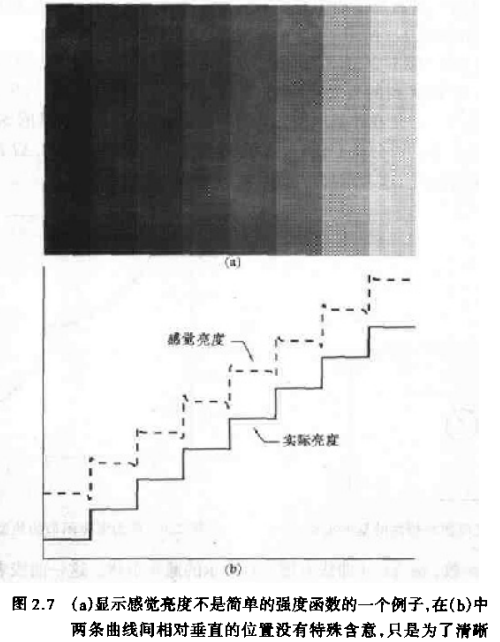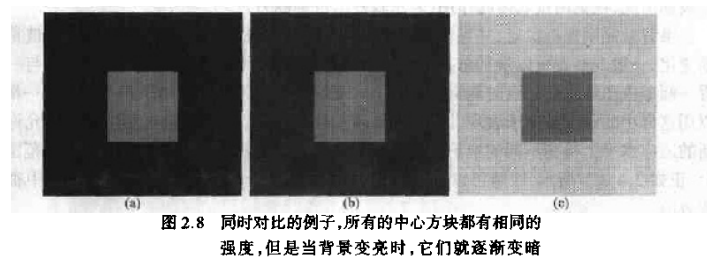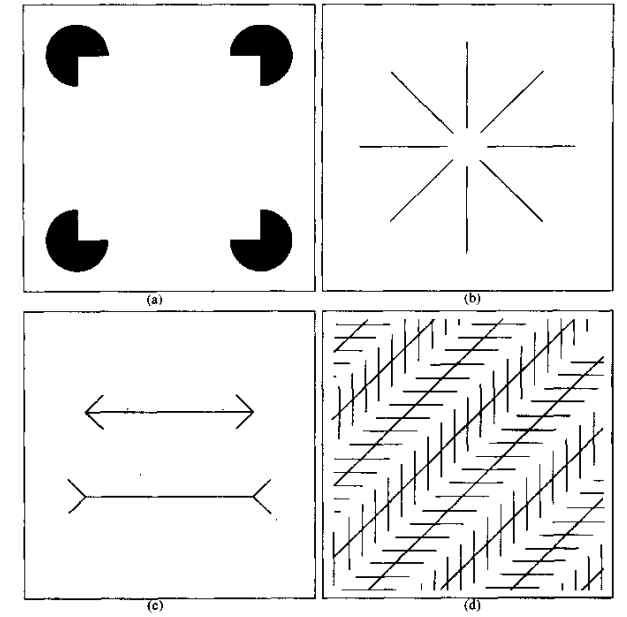# 开始学习数字图像处理

## 人类对图像亮度的理解

### 1. 视觉系统倾向于不同强度区域边界周围的“欠调”和“过调”### 2. 同时对比现象### 3. 视觉错觉λ=c/γ

E=hv

## 图像形成模型

0<f(x,y)<

f(x,y)=i(x,y)r(x,y),0<r(x,y)<1

i(x,y)表示入射分量，r(x,y)表示反射分量

## 像素表示形式

4领域：N4(p):{(x+1,y),(x-1,y),(x,y+1),(x,y-1)}
4对角领域：ND(p):{(x+1,y+1),(x+1,y-1),(x-1,y+1),(x-1,y-1)}
8领域：N8(p)

©️2019 CSDN 皮肤主题: 大白 设计师: CSDN官方博客